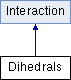ParM  parm A molecular dynamics library
Dihedrals Class Reference

#include <interaction.hpp>

Inheritance diagram for Dihedrals:## Public Member Functions

Dihedrals (vector< DihedralGrouping > pairs=vector< DihedralGrouping >())

void add (DihedralGrouping b)

void add (vector< flt > nums, AtomID a1, AtomID a2, AtomID a3, AtomID a4)

void add (vector< flt > cos_coefficients, vector< flt > sincoeffs, AtomID a1, AtomID a2, AtomID a3, AtomID a4, bool usepow=true)

void add (flt k, flt theta0, AtomID a1, AtomID a2, AtomID a3, AtomID a4)
Add 4 atoms with the potential $$V(\theta) = k (1 + \cos(\theta - \theta_0))$$. More...

void add (flt k, AtomID a1, AtomID a2, AtomID a3, AtomID a4)
Add 4 atoms with the potential $$V(\theta) = k (1 + \cos(\theta - \theta_0))$$, where $$\theta_0$$ is set to match the current positions of the atoms. More...

uint size () const

flt mean_dists () const

flt energy (Box &box)
Potential energy due to this Interaction. More...

void set_forces (Box &box)

flt pressure (Box &box)
Partial pressure due to this Interaction. More...

flt set_forces_get_pressure (Box &box)
Set forces (Atom.f) and return $$P = \sum_{\left<i,j \right>} \vec r_{ij} \cdot \vec F_{ij}$$ at the same time (see pressure()). More...Public Member Functions inherited from Interaction
virtual Matrix stress (Box &box)
The force-moment tensor for the current simulation: More...

virtual ~Interaction ()

## Protected Attributes

vector< DihedralGroupinggroups

## Constructor & Destructor Documentation

 Dihedrals::Dihedrals ( vector< DihedralGrouping > pairs = vector() )

## Member Function Documentation

 void Dihedrals::add ( DihedralGrouping b )
inline
 void Dihedrals::add ( vector< flt > nums, AtomID a1, AtomID a2, AtomID a3, AtomID a4 )
inline
 void Dihedrals::add ( vector< flt > cos_coefficients, vector< flt > sincoeffs, AtomID a1, AtomID a2, AtomID a3, AtomID a4, bool usepow = true )
inline
 void Dihedrals::add ( flt k, flt theta0, AtomID a1, AtomID a2, AtomID a3, AtomID a4 )
inline

Add 4 atoms with the potential $$V(\theta) = k (1 + \cos(\theta - \theta_0))$$.

 void Dihedrals::add ( flt k, AtomID a1, AtomID a2, AtomID a3, AtomID a4 )
inline

Add 4 atoms with the potential $$V(\theta) = k (1 + \cos(\theta - \theta_0))$$, where $$\theta_0$$ is set to match the current positions of the atoms.

 flt Dihedrals::energy ( Box & box )
virtual

Potential energy due to this Interaction.

Implements Interaction.

 flt Dihedrals::mean_dists ( ) const
 flt Dihedrals::pressure ( Box & box )
inlinevirtual

Partial pressure due to this Interaction.

$$P = \sum_{\left<i,j \right>} \vec r_{ij} \cdot \vec F_{ij}$$, or equivalently $$P = \sum_i \vec r_i \cdot \vec F_i$$

Note that the full pressure involves all interactions and temperature, and needs to be normalized by $$\frac{1}{dV}$$ where $$d$$ is the number of dimensions and $$V$$ is the volume.

Implements Interaction.

 void Dihedrals::set_forces ( Box & box )
virtual

Implements Interaction.

 flt Dihedrals::set_forces_get_pressure ( Box & box )
inlinevirtual

Set forces (Atom.f) and return $$P = \sum_{\left<i,j \right>} \vec r_{ij} \cdot \vec F_{ij}$$ at the same time (see pressure()).

Reimplemented from Interaction.

 uint Dihedrals::size ( ) const
inline

## Member Data Documentation

 vector Dihedrals::groups
protected

The documentation for this class was generated from the following files: